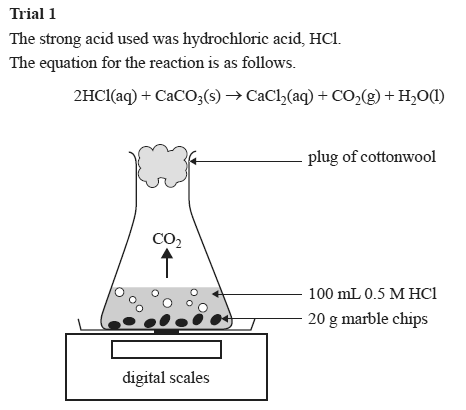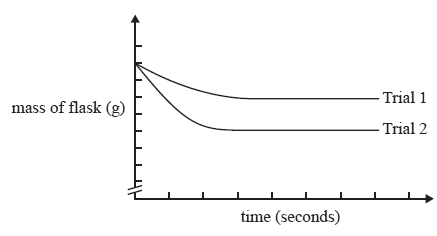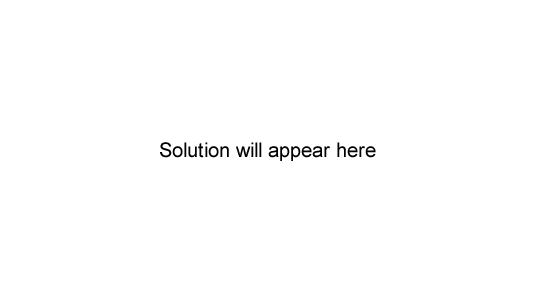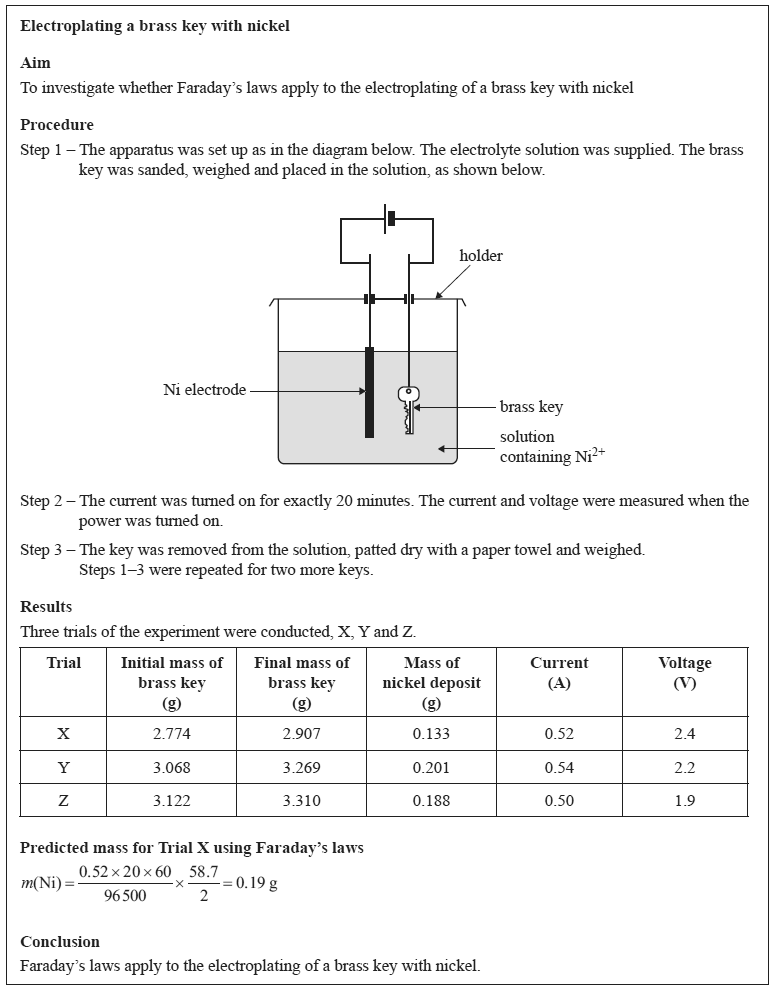Experimental technique 2016 VCE A student set up an experiment to test the effect of different factors on the rate and extent of the reaction between a strong acid and marble chips (calcium carbonate, CaCO3). In each trial, the mass of the flask and its contents was measured every 30 seconds, from the instant the reactants were mixed. Trial 1 The strong acid used was hydrochloric acid, HCl. The equation for the reaction is as follows. 2HCl(aq) + CaCO3(s) → CaCl2(aq) + CO2(g) + H2O(l)Trial 2 One change to the reactionconditions was made and the experiment was repeated. The results of the two trials were graphed on the same axes and are shown below.In Trial 2, the student must have A. heated the 0.5 M HCl before adding it to the flask. B. doubled the volume of 0.5 M HCl added to the flask. C. used 100 mL of 0.5 M H2SO4 instead of 100 mL of 0.5 M HCl. D. used the same mass of marble but crushed it into a powder.n is flawed   SolutionEvaluate the student's experimental design and report. In your response: • identify and explain one strength of the experimental design • suggest two improvements or modifications that you would make to the experimental design and justify your suggestions • comment on the validity of the conclusion based on the results obtained. Solution